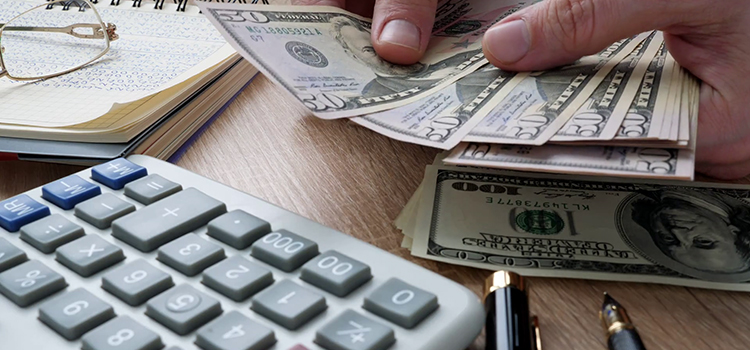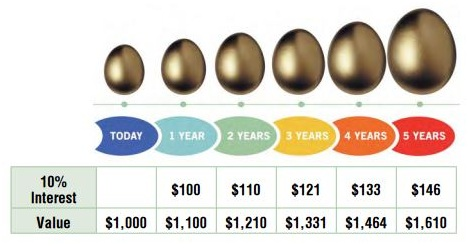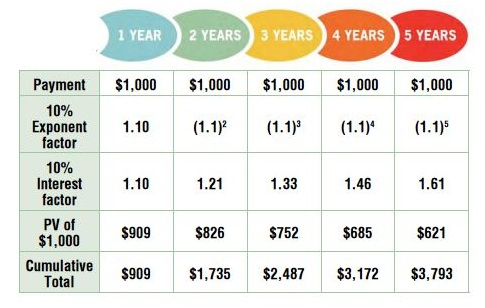# Calculating Present and Future Value in Divorce

You probably did not become a family lawyer because you loved to crunch numbers, but you should know how to perform basic present value and future value calculations. Here’s a primer for attorneys.

By Harriett Fox, Forensic Accountant

You probably became a lawyer for the exact opposite reasons that I became a CPA: I could not fathom doing all that reading and writing, and you probably could not fathom dealing with numbers. You did not become an attorney because you wanted to spend the rest of your life crunching numbers – but a lot of the work you do as an attorney requires some knowledge of calculations.

Almost every lawsuit includes a financial component. In a divorce, the financial components include valuing marital and non-marital assets and liabilities, determining each spouse’s income, and calculating spousal support (alimony) and child support. There may also be a closely-held business, stock options, or a pension that needs to be valued for inclusion in the marital estate. In non-family matters, the financial pieces can include calculating damages for insurance claims, personal injury, wrongful death, and tort actions to name a few.

A Grand Today is Better than a Grand Next Year

You can use that \$1,000 to make money between now and next year; if you can earn 10% interest, you will have \$1,100 a year from now. Put another way, the value of \$1,100 a year from now is equal to \$1,000 today. Expressed in financial terms, the present value of \$1,100 in one year, with a 10% discount (interest) rate, is \$1,000.

In divorces, the horizon is often longer than a year. For illustration purposes, we will use a five-year time-frame in our calculations. Using the same \$1,000 today, the following shows the future value of \$1,000 at 10% interest.

A Complicated Formula for an Uncomplicated ConceptIt is simple to use a mathematical expression to calculate future values. Instead of adding 10% interest each year, the factor of 1.1 is used to multiply the value of \$1,000 each year into the future. Calculating the value in 5 years at 10% interest is:

\$1,000 x (1.1) x (1.1) x (1.1) x (1.1) x (1.1).

Or, using exponents from algebra:

\$1,000 x (1.1)5

Expressed in financial terms:

Future Value (FV) = Present Value (PV) x (1 + interest rate (r))number of years (n)

Or FV = PV x (1 + r) n

Let’s look at an example of a future value calculation. If I buy a \$10,000 bond today that earns 10% interest with no payments until maturity, how much will the bond be worth in three years? The formula to calculate the future value is:

FV = PV x (1 + r) n

FV = \$10,000 x (1.1)3

FV = \$10,000 x 1.33

FV = \$13,300

Using this formula, it seems obvious that if the PV (the amount we start with) increases, then the FV will also increase. And, as long as the interest continues to accrue, the longer we hold the bond, the higher the FV of the bond will be. Finally, if the interest rate goes up, we will earn more interest on the initial \$10,000, and will, therefore, have a greater FV.

Reversing the Concept

Suppose instead of calculating the FV of a present amount, we want to find the PV of a payment in the future. Instead of multiplying the PV by an interest rate to calculate a FV, we divide the FV by a factor (discount rate) to calculate the PV.The factors are derived from the interest rate. For 10% interest, the factors for the first five years are as follows:

Since the formula for FV (from earlier) is: FV = PV x (1 + r) n

Using basic algebra, the PV is then: PV = FV / (1 + r) n

Let’s look at an example of a PV calculation. Suppose there is a marital certificate of deposit (CD), earning 10% interest, that matures in three years. It can be retitled from joint ownership to single ownership, but it cannot be redeemed without a large penalty. Rather than wait for the CD to mature in three years so the couple can divide the proceeds, one party will take the CD in exchange for some other marital asset. To ensure a fair exchange, we have to calculate what the value is today on a CD that matures in three years. The formula to calculate the PV is:

PV = FV / (1 + r) n

PV = \$10,000 / (1.1)3

PV = \$10,000 / 1.33

PV = \$7,518 (rounded)

Annual Payments in the Future

So far, we have presented cases that are for single payments in the future. What if there are annual payments? How can you find the PV of multiple future payments?

The basic calculation is the same. The PV of each year’s payment is calculated and summed to find the PV of the stream of payments. Suppose there are five annual payments of \$1,000. Each year’s payment would be divided by the factor for that year. The calculation looks like this:The PV of the five-year stream of payments is the sum of each of the values, or a total of \$3,793 for the five annual payments of \$1,000.

The Magic is in the Assumptions

Now that we know the formula for calculating PV, what could be controversial about the calculations?

Let’s see what happens when the basic variables are changed. The variables are:

PV – present value

FV – future value

r – interest rate

n – number of years in the future

Any one of the variables can be calculated when the other three values are known. The interest rate can be calculated if you know the PV, FV, and number of years. The number of years can be calculated knowing the PV, FV and interest rate. But we’re concerned with the PV calculation and how its factors impact the outcome.

Let’s use the example from the single payment PV calculation, changing the variables one at a time.

To review: we have to calculate what the value is today, on a 10% CD that matures in 3 years. This is the calculation from above.

PV = FV / (1 + r) n

PV = \$10,000 / (1.1)3

PV = \$10,000 / 1.33

PV = \$7,518 (rounded)

Now what happens when we change the variables?

Double the future value:

If the FV is \$20,000 instead of \$10,000, then the PV becomes:

PV = \$20,000 / (1.1)3

PV = \$20,000 / 1.33

PV = \$15,036 (rounded)

As expected, the PV increases proportionately as the FV increases. When we double the FV, the PV also doubles.

Double the number of years:

If the FV stays at \$10,000 and the number of years is 6 instead of 3, what is the PV?

PV = \$10,000 / (1.1)6

PV = \$10,000 / 1.77

PV = \$5,649 (rounded)

The PV of \$10,000 is less the further in the future we go. But it is not proportional. In other words, by doubling the time horizon, the PV was not reduced by half.

Double the interest rate:

If the FV is \$10,000 and the number of years is 3, what if the interest rate is 20% instead of 10%?

PV = \$10,000 / (1.2)3

PV = \$10,000 / 1.728

PV = \$5,787 (rounded)

The PV of \$10,000 is less than the resulting \$7,518 from the original scenario. But it is not proportional. In other words, by doubling the interest rate, the PV was not reduced by half.

Halve the interest rate:

If the FV is \$10,000 and the number of years is 3, what if the interest rate is 5% instead of 10%?

PV = \$10,000 / (1.05)3

PV = \$10,000 / 1.157

PV = \$8,643 (rounded)

The PV of \$10,000 is higher than the resulting \$7,518 from the original scenario. With a lower interest rate, the resulting PV is higher, but again, not proportionately.

The explanations contained here are just the tip of the PV iceberg; there are many applications for using PV calculations. Ask your financial professional to explain the elements of the PV calculations used in your cases. You should question the assumptions he/she is using – especially the interest rate (discount rate), as it has a major effect on the outcome. And you should always feel confident that your financial professional understands the calculations and isn’t pulling results out of a hat.

With an MBA from MIT and a Masters in Accounting, Harriett Fox (CPA) specializes in complex financial analyses. She has served as an expert witness in numerous cases, and she is a collaborative financial professional.www.harriettfoxcpa.com